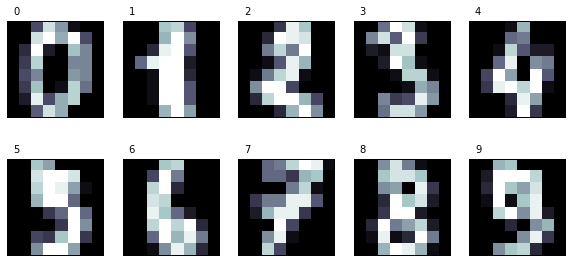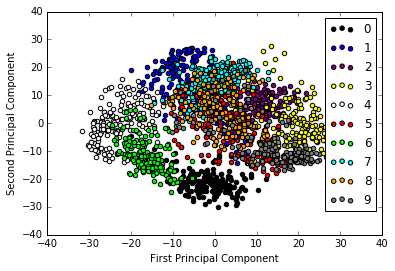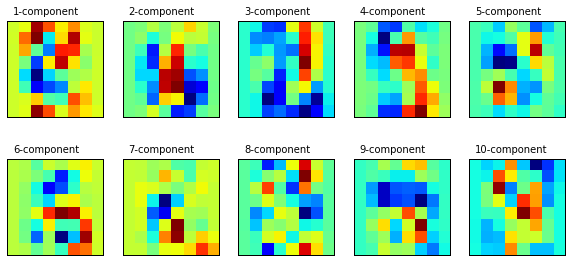# Principal Component Analysis (PCA)

is an orthogonal linear transformation that turns a set of possibly correlated variables into a new set of variables that are as uncorrelated as possible. The new variables lie in a new coordinate system such that the greatest variance is obtained by projecting the data in the first coordinate, the second greatest variance by projecting in the second coordinate, and so on. These new coordinates are called principal components; we have as many principal components as the number of original dimensions, but we keep only those with high variance. Each new principal component that is added to the principal components set must comply with the restriction that it should be orthogonal (that is, uncorrelated) to the remaining principal components. PCA can be seen as a method that reveals the internal structure of data; it supplies the user with a lower dimensional shadow of the original objects. If we keep only the first principal components, data dimensionality is reduced and thus it is easier to visualize the structure of data. If we keep, for example, only the first and second components, we can examine data using a two-dimensional scatter plot. As a result, PCA is useful for exploratory data analysis before building predictive models.

It is an unsupervised method since it does not need a target class to perform its transformations; it only relies on the values of the learning attributes.

We will use a dataset of handwritten digits digitalized in matrices of 8×8 pixels, so each instance will consist initially of 64 attributes. How can we visualize the distribution of instances?

In :
```%pylab inline
import numpy as np
import matplotlib.pyplot as plt
```
```Populating the interactive namespace from numpy and matplotlib
```
In :
```from sklearn.datasets import load_digits
X_digits, y_digits = digits.data, digits.target
```
In :
```print (digits.keys())
```
```dict_keys(['DESCR', 'data', 'target', 'target_names', 'images'])
```

We will use the data matrix that has the instances of 64 attributes each and the target vector that has the corresponding digit number.

Let us print the digits to take a look at how the instances will appear

In :
```n_row, n_col = 2, 5

def print_digits(images, y, max_n=10):
# set up the figure size in inches
fig = plt.figure(figsize=(2. * n_col, 2.26 * n_row))
i=0
while i < max_n and i < images.shape:
p = fig.add_subplot(n_row, n_col, i + 1, xticks=[], yticks=[])
p.imshow(images[i], cmap=plt.cm.bone, interpolation='nearest')
# label the image with the target value
p.text(0, -1, str(y[i]))
i = i + 1

print_digits(digits.images, digits.target, max_n=10)
```Function that will plot a scatter with the two-dimensional points that will be obtained by a PCA transformation. Our data points will also be colored according to their classes.

In :
```def plot_pca_scatter():
colors = ['black', 'blue', 'purple', 'yellow', 'white', 'red', 'lime', 'cyan', 'orange', 'gray']
for i in range(len(colors)):
px = X_pca[:, 0][y_digits == i]
py = X_pca[:, 1][y_digits == i]
plt.scatter(px, py, c=colors[i])
plt.legend(digits.target_names)
plt.xlabel('First Principal Component')
plt.ylabel('Second Principal Component')
```

In scikit-learn, PCA is implemented as a transformer object that learns n number of components through the fit method, and can be used on new data to project it onto these components. In scikit-learn, we have various classes that implement different kinds of PCA decompositions, such as PCA, ProbabilisticPCA, RandomizedPCA, and KernelPCA.

In our case, we will work with the PCA class from the sklearn.decomposition module. The most important parameter we can change is n_components, which allows us to specify the number of features that the obtained instances will have. In our case, we want to transform instances of 64 features to instances of just two features, so we will set n_components to 2.

In :
```from sklearn.decomposition import PCA

n_components = n_row * n_col
estimator = PCA(n_components=n_components)
X_pca = estimator.fit_transform(X_digits)
plot_pca_scatter()
```From the preceding figure, we can draw a few interesting conclusions:

• We can view the 10 different classes corresponding to the 10 digits at first sight. We see that for most classes, their instances are clearly grouped in clusters according to their target class, and also that the clusters are relatively distinct. The exception is the class corresponding to the digit 5 with instances very sparsely distributed over the plane overlap with the other classes.

• At the other extreme, the class corresponding to the digit 0 is the most separated cluster. Intuitively, this class may be the one that is easiest to separate from the rest; that is, if we train a classifier, it should be the class with the best evaluation figures.

• Also, for topological distribution, we may predict that contiguous classes correspond to similar digits, which means they will be the most difficult to separate. For example, the clusters corresponding to digits 9 and 3 appear contiguous (which will be expected as their graphical representations are similar), so it might be more difficult to separate a 9 from a 3 than a 9 from a 4, which is on the left-hand side, far from these clusters.

Let us look at principal component transformations. We will take the principal components from the estimator by accessing the components attribute. Each of its components is a matrix that is used to transform a vector from the original space to the transformed space. In the scatter we previously plotted, we only took into account the first two components.

We will plot all the components in the same shape as the original data (digits).

In :
```def print_pca_components(images, n_col, n_row):
plt.figure(figsize=(2. * n_col, 2.26 * n_row))
for i, comp in enumerate(images):
plt.subplot(n_row, n_col, i + 1)
plt.imshow(comp.reshape((8, 8)), interpolation='nearest')
plt.text(0, -1, str(i + 1) + '-component')
plt.xticks(())
plt.yticks(())
```
In :
```print_pca_components(estimator.components_[:n_components], n_col, n_row)
```If you look at the second component, you can see that it mostly highlights the central region of the image. The digit class that is most affected by this pattern is 0, since its central region is empty. This intuition is confirmed by looking at our previous scatter plot. If you look at the cluster corresponding to the digit 0, you can see it is the one that has the lower values for the second component.

• Regarding the first component, as we see in the scatter plot, it is very useful to separate the clusters corresponding to the digit 4 (extreme left, low value) and the digit 3 (extreme right, high value). If you see the first component plot, it agrees with this observation. You can see that the regions corresponding to the zone are very similar to the digit 3, while it has color in the zones that are characteristic of the digit 4.

excerpt from

This site uses Akismet to reduce spam. Learn how your comment data is processed.